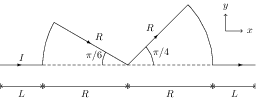# Force on a Current Carrying Wire in a Uniform Magnetic Field

## Problems from IIT JEE

Problem (IIT JEE 2015):A conductor (shown in the figure) carrying constant current $I$ is kept in the $x\text{-}y$ plane in a uniform magnetic field $\vec{B}$. If $F$ is the magnitude of the total magnetic force acting on the conductor, then the correct statement(s) is (are),

1. If $\vec{B}$ is along $\hat{z}$, $F\propto(L+R)$
2. If $\vec{B}$ is along $\hat{x}$, $F=0$
3. If $\vec{B}$ is along $\hat{y}$, $F\propto(L+R)$
4. If $\vec{B}$ is along $\hat{z}$, $F=0$

Solution:The force on a conducting element of length $\mathrm{d}\vec{l}$ carrying a current $I$ in a magnetic field $\vec{B}$ is given by $\mathrm{d}\vec{F}=I\,\mathrm{d}\vec{l}\times\vec{B}$. If the field is uniform, then the total force on the conductor is given by, \begin{align} \label{gyb:eqn:1} \vec{F}=\int_{a}^{g} I\,\mathrm{d}\vec{l}\times\vec{B}=I\left(\int_{a}^{g}\mathrm{d}\vec{l}\right)\times\vec{B}=I\;\stackrel{\to}{ag}\times\vec{B}. \end{align} Note that $\vec{B}$ is taken out of the integral because it is constant. The vector $\vec{ag}=2(L+R)\,\hat{x}$. The magnetic force on the conductor in the given cases are, \begin{align} &\text{Case (A):} &&\vec{F}=I(2(L+R)\,\hat{x})\times(B\hat{z})=-2IB(L+R)\,\hat{y},\nonumber\\ &\text{Case (B):} &&\vec{F}=I(2(L+R)\,\hat{x})\times(B\hat{x})=0,\nonumber\\ &\text{Case (C):} &&\vec{F}=I(2(L+R)\,\hat{x})\times(B\hat{y})=2IB(L+R)\,\hat{z}.\nonumber % &\text{Case (D):} &&\vec{F}=I(2(R+L)\,\hat{x})\times(B\hat{z})=-2IB(R+L)\,\hat{y},\nonumber \end{align} Thus, correct options are A, B, and C.